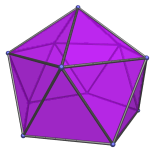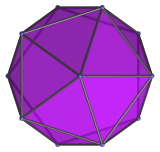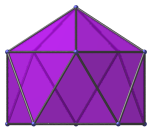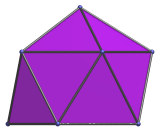# The Gyroelongated Pentagonal Pyramid

The gyroelongated pentagonal pyramid is the 11th Johnson solid (J11). It has 11 vertices, 25 edges, and 16 faces (15 equilateral triangles and 1 pentagon).The gyroelongated pentagonal pyramid can be constructed by attaching a pentagonal pyramid to a pentagonal antiprism. It can also be constructed by deleting a single vertex from the icosahedron, and so can also be called the monodiminished icosahedron. It can be further diminished by removing a vertex not opposite the pentagonal face, producing a metabidiminished icosahedron (J62), or by removing two vertices, producing a tridiminished icosahedron (J63).

## Projections

Here are some views of the gyroelongated pentagonal pyramid from various angles:

Projection Envelope DescriptionRegular decagon

Top view.Pentagon

Side view.Pentagon

Front view.

## Coordinates

The Cartesian coordinates of the gyroelongated pentagonal pyramid with edge length 2 are:

• (0, 0, √((5+√5)/2))
• ±(B, 0, A)
• ±(C, ±φ, A)
• ±(−D, ±1, A)

where

 A = √((5+√5)/10) B = √((10+2√5)/5) C = √((5−√5)/10) D = √((5+2√5)/5) φ = (1+√5)/2

Last updated 18 Jun 2019.